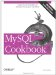# Calculating Intervals Between Dates

5.18.1 Problem

You want to know how long it is between dates.

5.18.2 Solution

Convert both dates to basic units and take the difference between the resulting values.

5.18.3 Discussion

The general procedure for calculating an interval between dates is to convert both dates to a common unit in relation to a given reference point, then take the difference. The range of values you're working with determines which conversions are available. DATE, DATETIME, or TIMESTAMP values dating back to 1970-01-01 00:00:00 GMTthe date of the Unix epochcan be converted to seconds elapsed since the epoch. If both dates lie within that range, you can calculate intervals to an accuracy of one second. Older dates from the beginning of the Gregorian calendar (1582) on can be converted to day values and used to compute intervals in days. Dates that begin earlier than either of these reference points present more of a problem. In such cases, you may find that your programming language offers computations that are not available or are difficult to perform in SQL. If so, consider processing date values directly from within your API language. (For example, the Date::Calc and Date::Manip modules are available from the CPAN for use within Perl scripts.)

To calculate an interval in days between date or date-and-time values, convert them to days using TO_DAYS( ), then take the difference:

```mysql> SELECT TO_DAYS('1884-01-01') - TO_DAYS('1883-06-05') AS days;
+------+
| days |
+------+
| 210 |
+------+```

For an interval in weeks, do the same thing and divide the result by seven:

```mysql> SELECT (TO_DAYS('1884-01-01') - TO_DAYS('1883-06-05')) / 7 AS weeks;
+-------+
| weeks |
+-------+
| 30.00 |
+-------+```

You cannot convert days to months or years by simple division, because those units vary in length. Calculations to yield date intervals expressed in those units are covered in Recipe 5.20.

## Do You Want an Interval or a Span?

Taking a difference between dates gives you the interval from one date to the next. If you want to know the range covered by the two dates, you must add a unit. For example, it's three days from 2002-01-01 to 2002-01-04, but together they span a range of four days. If you're not getting the results you expect from an interval calculation, consider whether you need to use an "off-by-one" correction.

For values occurring from the beginning of 1970 on, you can determine intervals to a resolution in seconds using the UNIX_TIMESTAMP( ) function. For example, the number of seconds between dates that lie two weeks apart can be computed like this:

```mysql> SET @dt1 = '1984-01-01 09:00:00';
mysql> SET @dt2 = '1984-01-15 09:00:00';
mysql> SELECT UNIX_TIMESTAMP(@dt2) - UNIX_TIMESTAMP(@dt1) AS seconds;
+---------+
| seconds |
+---------+
| 1209600 |
+---------+```

To convert the interval in seconds to other units, perform the appropriate arithmetic operation. Seconds are easily converted to minutes, hours, days, or weeks:

```mysql> SET @interval = UNIX_TIMESTAMP(@dt2) - UNIX_TIMESTAMP(@dt1);
mysql> SELECT @interval AS seconds,
-> @interval / 60 AS minutes,
-> @interval / (60 * 60) AS hours,
-> @interval / (24 * 60 * 60) AS days,
-> @interval / (7 * 24 * 60 * 60) AS weeks;
+---------+---------+-------+------+-------+
| seconds | minutes | hours | days | weeks |
+---------+---------+-------+------+-------+
| 1209600 | 20160 | 336 | 14 | 2 |
+---------+---------+-------+------+-------+```

For values that occur prior outside the range from 1970 to 2037, you can use an interval calculation method that is more general (but messier):

• Take the difference in days between the date parts of the values and multiply by 24*60*60 to convert to seconds.
• Offset the result by the difference in seconds between the time parts of the values.

Here's an example, using two date-and-time values that lie a week apart:

```mysql> SET @dt1 = '1800-02-14 07:30:00';
mysql> SET @dt2 = '1800-02-21 07:30:00';
mysql> SET @interval =
-> ((TO_DAYS(@dt2) - TO_DAYS(@dt1)) * 24*60*60)
-> + TIME_TO_SEC(@dt2) - TIME_TO_SEC(@dt1);
mysql> SELECT @interval AS seconds;
+---------+
| seconds |
+---------+
| 604800 |
+---------+```

To convert the interval to a TIME value, pass it to SEC_TO_TIME( ):

```mysql> SELECT SEC_TO_TIME(@interval) AS TIME;
+-----------+
| TIME |
+-----------+
| 168:00:00 |
+-----------+```

To convert the interval from seconds to other units, perform the appropriate division:

```mysql> SELECT @interval AS seconds,
-> @interval / 60 AS minutes,
-> @interval / (60 * 60) AS hours,
-> @interval / (24 * 60 * 60) AS days,
-> @interval / (7 * 24 * 60 * 60) AS weeks;
+---------+---------+-------+------+-------+
| seconds | minutes | hours | days | weeks |
+---------+---------+-------+------+-------+
| 604800 | 10080 | 168 | 7 | 1 |
+---------+---------+-------+------+-------+```

I cheated here by choosing an interval that produces nice integer values for all the division operations. In general, you'll have a fractional part, in which case you may find it helpful to use FLOOR(expr) to chop off the fractional part and produce an integer.MySQL Cookbook
ISBN: 059652708X
EAN: 2147483647
Year: 2005
Pages: 412
Authors: Paul DuBois